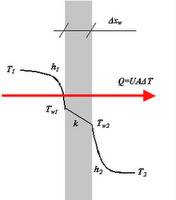How to calculate the time and distance needed to heat an object

Hi,

I have a small rubber object that I want to heat to a particular temperature. I have done some research and I have found a formula to determine the heat capacity that I need in order to reach the specified temperature.

However, the formula does not tell me :

1.) How long will it take to reach the temperature?

2.) What distance does the source of the heat need to be from the target of the heat?

I would like to know how I can calculate both 1 and 2?

Much appreciated,
--thepok

What formula is that? Heat capacity of an object is the amount of heat that is needed to raise the temperature of the object by 1 degree kelvin (or celcius)

The easiest way of to get the right temperature would be to put in in a water bath, or oven with the temperature controlled by a thermostat.

What formula is that?

q = mc (Tf-Ti)

It roughly came to about 1100 Joules

Heat capacity of an object is the amount of heat that is needed to raise the temperature of the object by 1 degree kelvin (or celcius)

Are you saying that I need 1100 Joules in order to raise the temperature of the object with 1 degree celcius? Or does the formula mean that I need 1100 Joules in order to raise the temperature to the desired temperature?

It would seem that I could use Newton's law of cooling to calculate the time it would take to heat the object.

The formula is only valid if you can make sure that all your energy goes into the object that you want to heat, like heating a liquid in a thermosflask with electric current.
This is probably the only case where you can get an accurate answer.

One problem with heating a rubber object, is that the heat conductivity of rubber is
low, so you'll have to heat the rubber very slowly if you don't want to overheat the outside. Newton's law of cooling is probably not very useful, because it's valid for an
object that has a single temperature.

QuantumPion
Gold Member
Last edited:
Remember the rate at which the object will reach a uniform equilibrium temperature is dependent on many factors.

One of which is the shape of the object

A rubber cube will take longer than a rubber strip that has the same mass (more surface area)

You need to determine the surrouding conditions and the heat transfer coefficients so as to get an estimate of the Overall Heat Transfer Coefficient (U). Q=UA[T-To]. So UA[T-To] = mCDT

Knowing the Heat Capacity which is simply a thermal property of the solid material is insufficient to answer your question.

Treat it like a standard heat transfer or heat exchange problem between two substancesand this may be a useful analogy
http://www.picotech.com/experiments/heat_transfer_coefficient/graphics/heat_der.gif

Last edited: